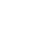Guide to Graphical Projections

Graphical Projections

Graphical projections are a set of rules to represent a 3D object in 2D space. Why is it important? Well, understanding it is an ideal fundamental to learning about projections or 3d graphics. The idea of this blog is to familiarise with different graphical projections that exist.

Graphical projections are achieved with the help of an imaginary projector that projects the 3d object to a planar surface. There are two categories of such rules or protocols:

• Parallel Projections
• Perspective Projections

Let us delve into the details of both.

Parallel Projection

For the rest of the article, let us assume the projector is our eye, unless specified otherwise. So the basis of all for parallel projection is, the rays/lines from the projector to the plane are parallel.

This would require some imagination, since our normal sight is converging. The following image is to better assist you.The rays from the object are parallel as they proceed to intersect at the projection plane.

Parallel projection have different sub-types, we will go through each of them briefly.

Orthographic Projection

This probably is the most popular parallel projection, since it is used in technical drawings a lot. In this parallel projection, the rays or projection lines intersect the plane orthogonally or simply at 90ºs or right angles. To get a comprehensive ideas about the object being represented we need views from different directions, from front, top and the sides.Pictorials

Idea of pictorials is to represent all the three axes of the object in a single image, unlike orthographic projection which requires three images to achieve this. To meet this end, the object is viewed from a skew direction. Simply put, we see the object from a position that is neither right angle nor parallel to the object being projected.

There are different kinds of pictorials:

Axonometric Projection

In axonometric projection as name suggest, we rotate the 3d object along one of the three axes, x, y or z. Axonometric projection have three sub-categories:

1. Isometric Projection

We position the object in such a way that, all side are equally distant from the axes and axes have a command angle of 120º between each.2. Diametric Projection

Like Isometric, but only the axes of object is only equidistant from two standard axes, and there is a 120º angle between those axes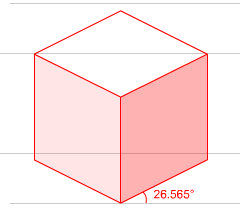3. Trimetric Projection

Well, the simple explanation would be all axes will be unequally spaced, and depending upon the angle of viewing, the angle between the object’s axes are determined. An image of all the three together will help you better understand trimetric projection.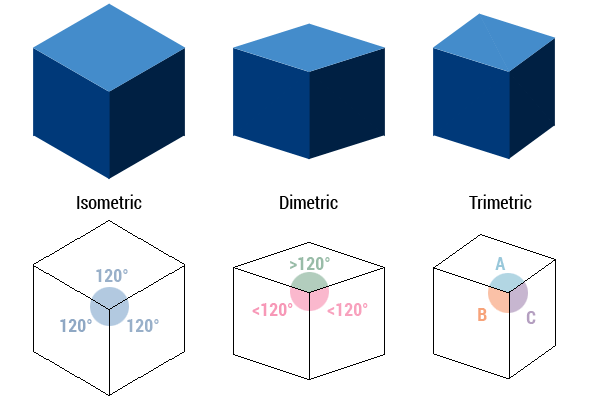Oblique Projection

It is the next sub-type of parallel projection. Here the governing concept is that the rays are not perpendicular to the plane on which we do projection. It is normally used for pictorial representation than for formal purposes.

Cavalier & Cabinet Projections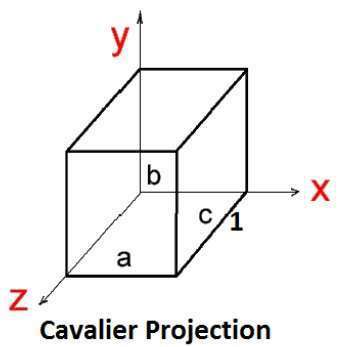Cavalier projection or Cabinet projection is probably one you have seen in your schools when the teacher tries to explain 3d geometry. Well in both the cases, there are two axes that will be mutually perpendicular to each other, as shown in the figure. And they will be drawn at a 1:1 scale.

The difference between these to projections are the angle they make with the projection plane.  It is 45º with projection plane for Cavalier projection. While cabinet projection makes 63.4º.

Military ProjectionThe difference of military projection is, all the three axes are perpendicular to each other,  The x-z plane is rotated by 45º this make the plan appear not skewed.

Perspective ProjectionPerspective projection is the most relevant projection for us. We will be just discussing the idea of this projection briefly and will set apart another blog post entirely for the details of this projection.

The idea behind perspective projection is, it is the projection as seen through a human eye. It converge at our eye and diverges as it go towards infinity.

Hope you have a good idea of what projections are, details about different graphical projections.

Send Lesson #1
Join 1000s of VR Developers

Build your Career in VR with this 6 part FREE course

Send Lesson #1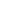Add Me To VIP
Join 1000s of VR Developers

We've build a simple curriculum to help you get started in VR

Send Lesson #1We've launched a WebVR Course with 25+ projects with source code! Check it out.